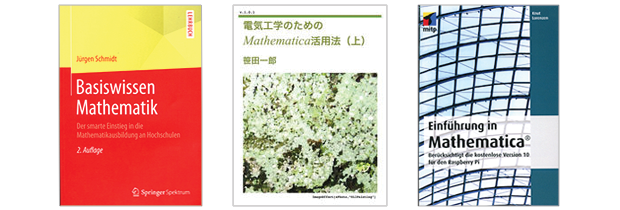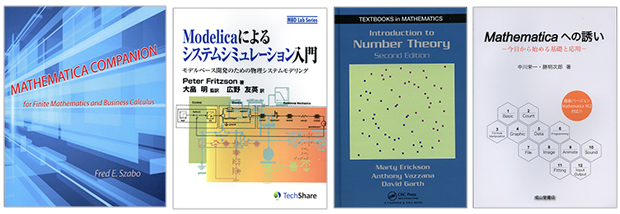Browse by Topic
Related Topics

# New Wolfram Technologies Books Reach a Global Audience

As this new year begins and the books keep rolling in, we are happy to share with you an exciting new selection of texts featuring Wolfram technologies. If you’re looking for a New Year’s resolution for 2016, why not consider learning how to use Mathematica or the Wolfram Language? In this post are several books for beginners in English, German, and Japanese, as well as more advanced books for those who are looking to sharpen their skills.Basic Mathematics Knowledge: The Smart Start to University Math (German)

This resource, written by Jürgen Schmidt for students minoring in mathematics, clearly explains key mathematical concepts. Multiple examples, an extensive practice section, and inclusion of Wolfram Research’s free “knowledge engine,” Wolfram|Alpha, provide help and orientation to students who study math at the college level. High-school graduates preparing for a career in the sciences, engineering, or economics, as well as students wishing to keep up with math, will appreciate the included collection of annotated formulas.

How to Utilize Mathematica in the Field of Electrical Engineering (Japanese)

Ichiro Sasada’s book picks out the basic tasks in the common areas of electrical engineering—electrical mathematics, electrical circuits, electromagnetism, and digital signal processing—and describes in detail how to use Mathematica to solve them. Each step includes code that can be used in the calculations. The objective of this book is to help students utilize the power of Mathematica, which could be called “state-of-the-art paper and pencil,” and to help them understand the problems in electrical engineering more quickly and deeply.

Introduction to Mathematica: Including the Free Version 10 for Raspberry Pi (German)

After a brief introduction to Mathematica, this book by Knut Lorenzen gives an overview of Mathematica’s functionalities and of the features of the knowledge base Wolfram|Alpha. Topics covered include options, numerical output, constants, units, types of numbers, fractions, power series, and free-form natural language input. Other chapters discuss lists, tables, expressions, functions, and user-defined objects, as well as graphic and sound features and music. Readers will learn the fundamental principles of programming with Mathematica and the Wolfram Language and how to use Mathematica with the Raspberry Pi.Mathematica Companion for Finite Mathematics and Business Calculus

This companion, written by Fred Szabo, is meant as a reference to many of the basic concepts and formulas of finite mathematics and calculus required in business and economics as well as in the life and social sciences. The material is organized and presented in a dictionary-style format. All concepts and formulas are illustrated with examples written in the Wolfram Language, the foremost computational software system used in scientific, engineering, mathematical, and computing fields. Throughout the book, the emphasis is on how to solve mathematical problems and to learn and explore mathematical ideas with Mathematica and Wolfram|Alpha.

Introduction to System Simulation with Modelica (Japanese)

This book is the translated version of Introduction to Modeling and Simulation of Technical and Physical Modeling with Modelica (Wiley, 2011), written by Professor Peter Fritzson, a board member of the Modelica Association. It details the basics of physical modeling with Modelica, the increasingly popular physical modeling language. The book covers the meaning of modeling, the purpose of modeling, common problems, and the categorization of modeling. It is ideal for those who are new to Modelica or want to try it.

Introduction to Number Theory, 2nd Edition

In this second edition, Martin Erickson, Anthony Vazzana, and David Garth provide a classroom-tested, student-friendly text that covers a diverse array of number theory topics, from the ancient Euclidean algorithm for finding the greatest common divisor of two integers to recent developments such as cryptography, the theory of elliptic curves, and the negative solution of Hilbert’s tenth problem. The authors illustrate the connections between number theory and other areas of mathematics and describe applications of number theory to real-world problems. This text provides calculations for computational experimentation using Mathematica and other popular software packages online via a robust, author-maintained website, and includes a solutions manual.

Introduction to Mathematica—Basics and Applications (Japanese)

Eiichi Nakagawa, a professor emeritus, and Akijiro Katsu, a Wolfram Training certified instructor, put together their extensive knowledge of Mathematica in this hands-on reference book that introduces Mathematica 10.2. This book consists of two chapters, one covering Mathematica basics and the other covering its applications. It is ideal for beginners looking to improve their skills. A notebook in the second chapter can be downloaded to the user’s computer.

Looking for more Wolfram technologies books? Don’t forget to visit Wolfram Books to browse by both topic and language!